Search the World's Largest Database of Information Science & Technology Terms & Definitions
InfScipedia
A Free Service of IGI Global Publishing House
Below please find a list of definitions for the term that
you selected from multiple scholarly research resources.

# What is Decision Tree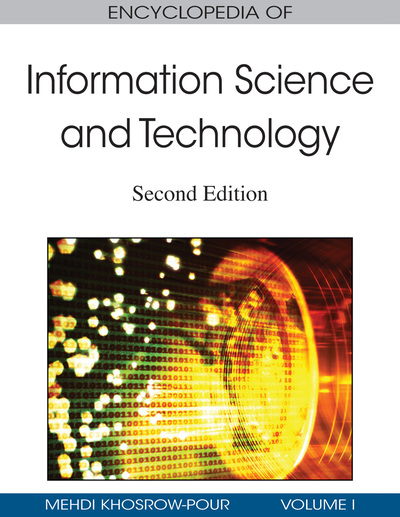A symbolic learning classifier that represents a discrete function by a decision tree.
Published in Chapter:
Machine Learning
João Gama (University of Porto, Portugal) and André C.P.L.F. de Carvalho (University of São Paulo, Brazil)
DOI: 10.4018/978-1-60566-026-4.ch392
Abstract
Machine learning techniques have been successfully applied to several real world problems in areas as diverse as image analysis, Semantic Web, bioinformatics, text processing, natural language processing,telecommunications, finance, medical diagnosis, and so forth. A particular application where machine learning plays a key role is data mining, where machine learning techniques have been extensively used for the extraction of association, clustering, prediction, diagnosis, and regression models. This text presents our personal view of the main aspects, major tasks, frequently used algorithms, current research, and future directions of machine learning research. For such, it is organized as follows: Background information concerning machine learning is presented in the second section. The third section discusses different definitions for Machine Learning. Common tasks faced by Machine Learning Systems are described in the fourth section. Popular Machine Learning algorithms and the importance of the loss function are commented on in the fifth section. The sixth and seventh sections present the current trends and future research directions, respectively.
More Results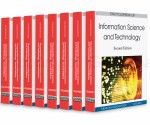A visual representation of a decision problem that forms tree-like predictive models with the aim of helping people to make better decisions.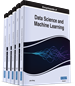A decision tree is a decision-making aid that employs a tree-like model of choices and their potential results, such as chance event outcomes, cost objects, and usefulness. It’s one approach to show an algorithm made up entirely of conditional control statements.A decision tree is a tree like model of decisions. In decision tree each branch represents the outcome of the test.A tree like structure for modeling decisions and classifying data.A diagram consisting of square decision nodes, circle probability nodes, and branches representing decision alternatives.A graphical representation for a decision problem that specifies the decisions that must be made, the chance events the decision maker faces, and the possible outcomes that result from the decisions and chance events that occurs.A decision tree is a graph that uses a branching method to illustrate every possible outcome of a decision. Each branch of the decision tree represents a possible decision or occurrence. The tree structure shows how one choice leads to the next, and the use of branches indicates that each option is mutually exclusive.A tree like structure for modeling decisions and classifying data.A tool to help make decisions based on a set of rules that help to navigate the tree along its branches.Is a decision support tool that uses a tree-like graph or model of decisions and their possible consequences, including chance event outcomes, resource costs, and utility.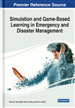A diagram showing branching paths through various action sequences based on decisions made at various junctures.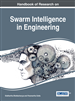Decision support method that uses a tree-like graph with algorithm for partitioning.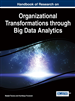A tree like structure that is used for classification of different records.A decision tree consists of a root node, representing a decision, a set of intermediate (event) nodes, representing some kind of uncertainty and consequence nodes, representing possible final outcomes. Usually, probability distributions are assigned in the form of weights in the probability nodes as measures of the uncertainties involved.A schematic tree-shaped diagram used to determine a course of action or show a statistical probability. Each branch of the decision tree represents a possible decision or occurrence.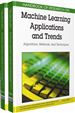A classifier in the form of a tree structure, where each node is either a leaf node or a decision node. A leaf node indicates the value of the target attribute (class) of examples.It is a tree-like model that is used for making the decision.Intelligent model based on rules and used to predict.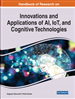Is a flowchart-like structure in which each internal node represents a “test” on an attribute. i.e., whether a coin flip comes up heads or tails, each branch represents the outcome of the test, and each leaf node represents a class label. Decision taken after computing all attributes.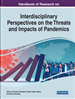A classification method that has the appearance of a tree structure.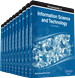A tree like structure for modeling decisions and classifying data.A decision analysis method that, on the basis of the known probability of various situations, calculates the probability of the expected value of the net present value being greater than or equal to zero by constructing the decision tree, evaluates the project risk, and determines its feasibility.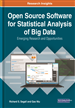Decision tree is a decision support tool that uses tree-like model to explore the possible outcomes.Decision trees are useful for topics that can be given in the form of unit answers such as category or class nameBuilds classification models in the form of a tree structure by breaking down a dataset into smaller and smaller subsets, while at the same time, an associated decision tree is incrementally developed. The final result is a tree with decision nodes (having two or more branches) and leaf nodes (representing a classification or decision).A predictive model which can be visualized in a hierarchical structure using leaves and ramifications.A decision tree is a graph that uses a branching method to illustrate every possible outcome of a decision. Each branch of the decision tree represents a possible decision or occurrence. The tree structure shows how one choice leads to the next, and the use of branches indicates that each option is mutually exclusive.It is amodel where sampling is divided based on the level of the tree, and the mean as well as standard deviation of the target variable are determined at the leaf nodes.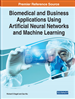A method that build a tree-like models to present all the possible consequences in different kinds of data is used in the process of data prediction.Refers to a tree-shaped model used to make decisions, with each internal node representing a “test” on an attribute, and each leaf node representing a class label.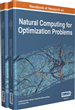A graphical representation or plot that reflects the initial states, evolution and possible outcome of a CA that is guided by specified rules.A tool to help make decisions based on a set of rules that help to navigate the tree along its branches.this is a scheme in which the person decides according to the given possibilities and then proceeds to the goal to which he/she had established his / her previous selections/decisions.A decision tree is a graph that uses a branching method to illustrate every possible outcome of a decision. Each branch of the decision tree represents a possible decision or occurrence. The tree structure shows how one choice leads to the next, and the use of branches indicates that each option is mutually exclusive.A tree-like way of representing a collection of hierarchical decision rules that lead to a class or value, starting from a root node ending in a series of leaf nodes.It is graphical illustration of sequence of actions which result in utility or loss.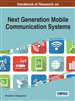Is a decision support tool that uses a tree-like graph or model of decisions and their possible consequences, including chance event outcomes, resource costs, and utility. It is one way to display an algorithm.It is technique for classifying data. The root node of a decision tree represents all examples. If these examples belong to two or more classes, then the most discriminating attribute is selected and the set is split into multiple classes.A rooted, ordered, and labeled tree. Given a boolean function on n-variables (tests), a decision tree is a deterministic algorithm for deciding which variable to test next, based on the previously tested variables and the results of their evaluation, until the function’s value can be determined.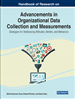A machine learning algorithm that cascades data through a series of sequential decisions to determine classification or regression outcomes.A tree-like structure for representing a collection of hierarchical decision rules that lead to a class or value, starting from a root node ending in a series of leaf nodes.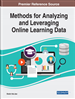A predictive model induced from quantitative and qualitative (nominal, categorical) data.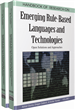is intuitive way for specifying decision procedures, a simple visual representation (an acyclic, directed graph).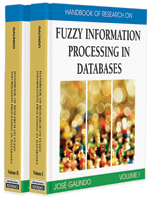It is a tree-like way of representing a collection of hierarchical decision rules that leads to a class or value, starting from a root node ending in a series of leaf nodes.A decision tree is a graph that uses a branching method to illustrate every possible outcome of a decision. Each branch of the decision tree represents a possible decision or occurrence. The tree structure shows how one choice leads to the next, and the use of branches indicates that each option is mutually exclusive.